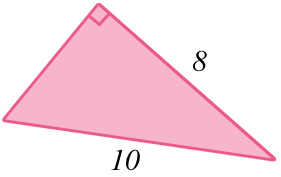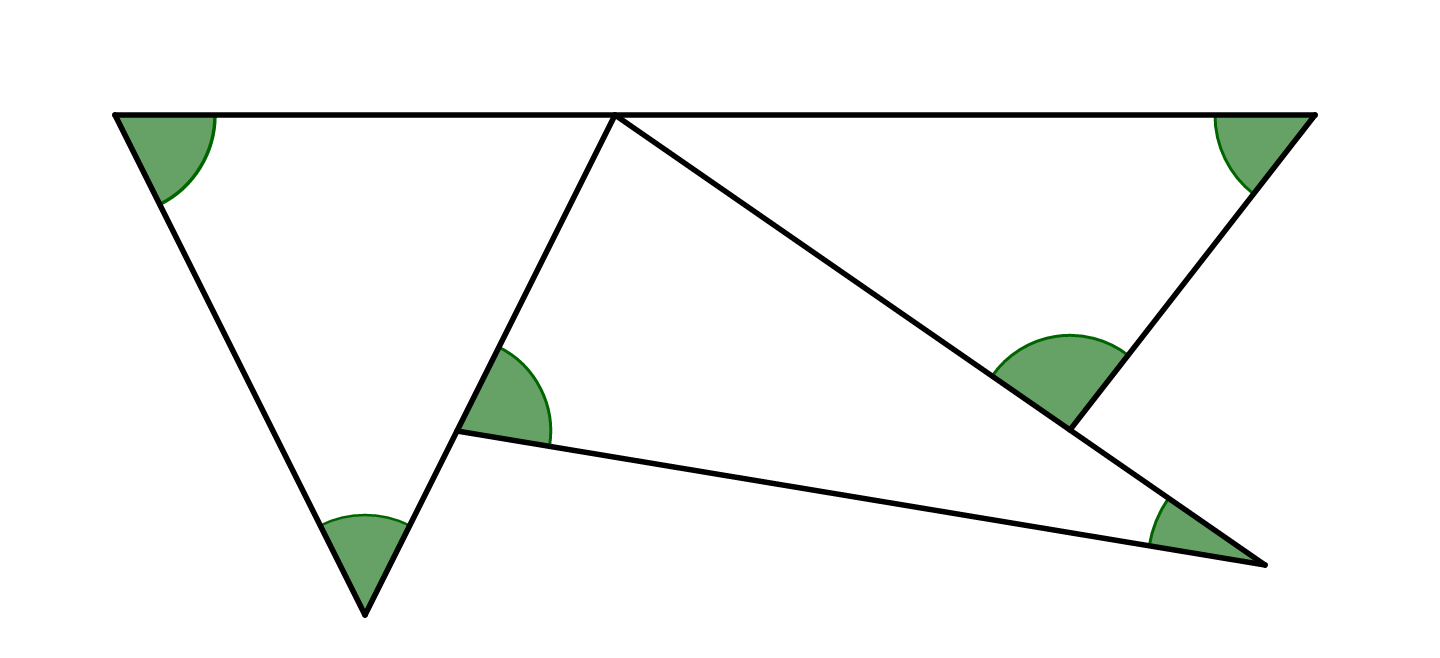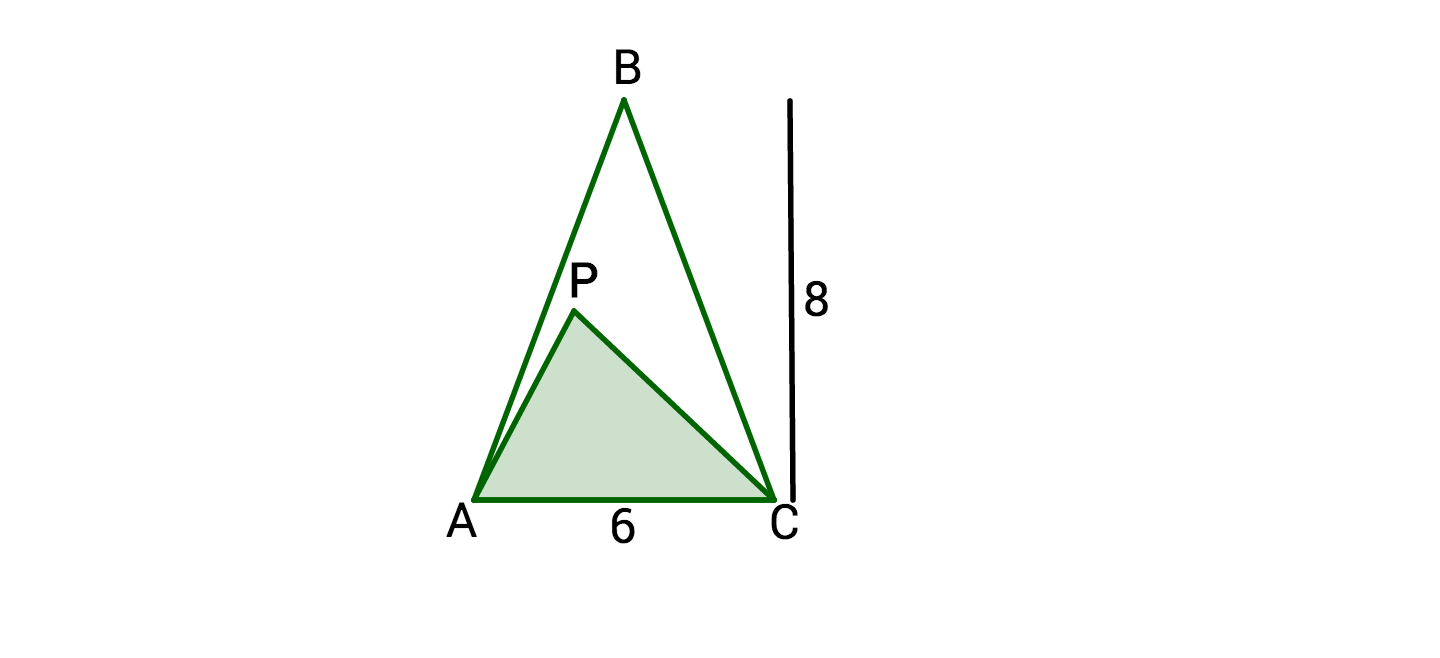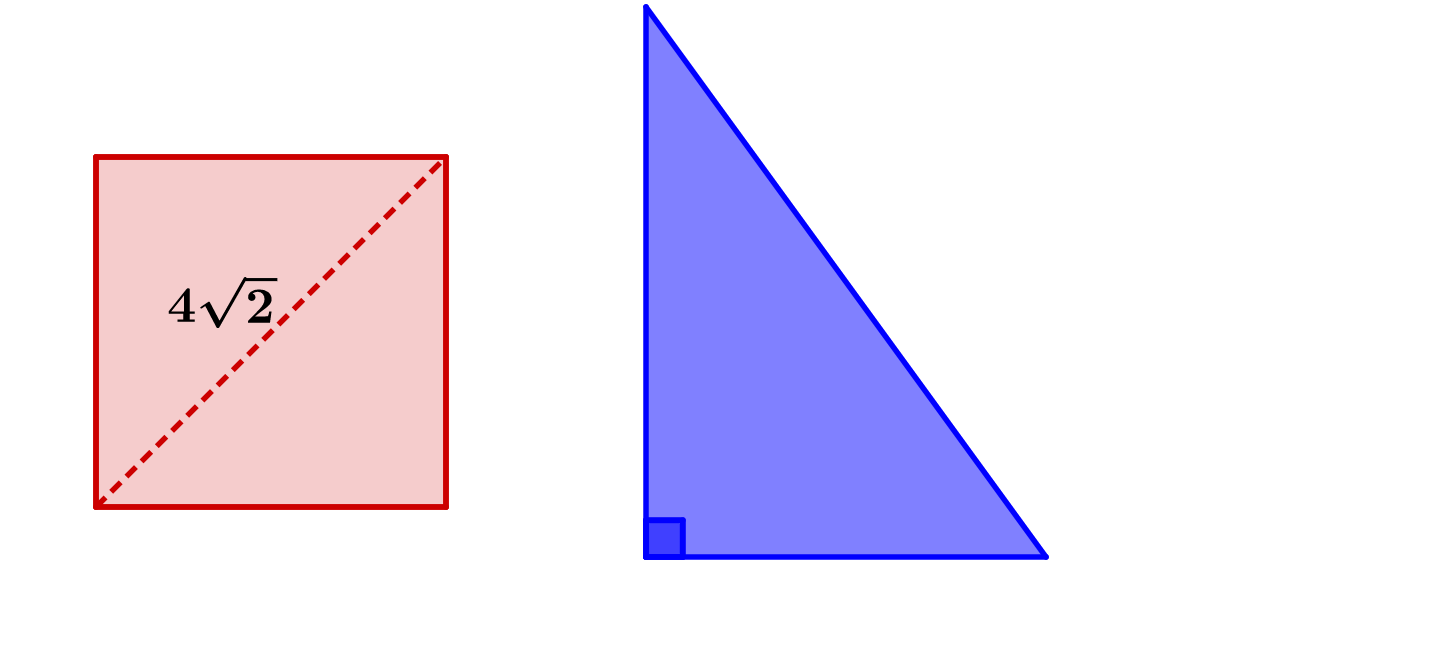Geometry

# Properties of Triangles WarmupWhat is the area of the triangle?What is the sum of the angles shaded in green?If the height of $$\triangle APC$$ is 4, what percent of $$\triangle ABC$$ is shaded white?If a square with a diagonal length of $$4\sqrt{2}$$ has the same area as a right triangle, what could be the base and height of the triangle?

If a right triangle has two side lengths of 1 and 2, which of these options couldn't be the length of the third side?

×

Problem Loading...

Note Loading...

Set Loading...